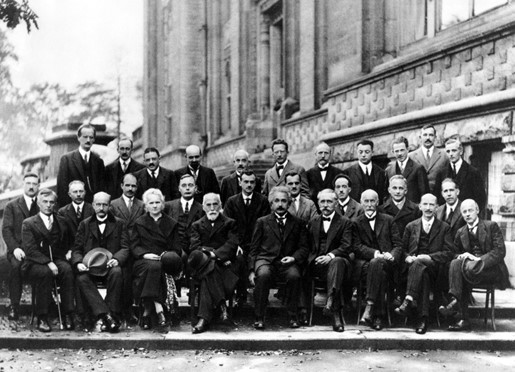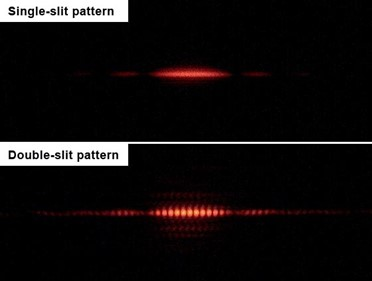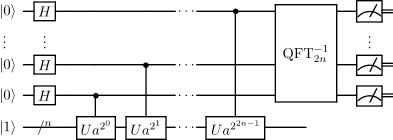# V. The Nature of Quantum Mechanics – Infinite, Non-Local, Computing Capacity

“It is impossible that the same thing belong and not belong to the same thing at the same time and in the same respect.”; “No one can believe that the same thing can (at the same time) be and not be.”; “The most certain of all basic principles is that contradictory propositions are not true simultaneously.” – Aristotle’s Law of Non-Contradiction, “Metaphysics (circa 350 B.C.) Via Wikipedia

Max Planck in 1900, in order to solve the blackbody radiation problem, and Albert Einstein in 1905, to explain the photoelectric effect, postulated that light itself was made of individual “energy quanta” and so began the theory of quantum mechanics. In the early 20th century many titans of physics would contribute to this strange theory, but a rare, rather intuitive, discovery occurred in 1948 when Richard Feynman invented a tool called the path integral. When physicists wanted to calculate the probability that, say, an electron, travels from A to B they used the path integral. The path integral appears as a complex exponential function like$e^{-i\Phi(x)}$ in physics equations, but this can be conceptually understood simply as a two-dimensional wave because:$e^{-i\Phi(x)}=cos\Phi(x)+isin\Phi(x)$

The real component represents one direction (e.g. horizontal-axis), while the other, “imaginary”, component another (e.g. vertical-axis). This complex function in the path integral, and in quantum mechanics in general, just means the wave is two-dimensional, not one. Think of a rope with one person holding each end. A vertical flick by one person sends a vertical wave propagating along the rope toward the other – this is not the path integral of quantum mechanics. Neither is a horizontal flick. Instead, imagine making a twisting flick, both vertical and horizontal. A corkscrew shaped wave propagates down the rope. This two-dimensional wave captures the nature of quantum mechanics and the path integral, but the wave is not known to be something physical like the wave on the rope. It is, rather, a wave of probability (a.k.a. a quantum wave function).Figure 13: The titans of quantum physics -1927 Solvay Conference on Quantum Mechanics by Benjamin Couprie via Wikimedia Commons.

The path integral formulation of quantum mechanics is mathematically equivalent to the Schrödinger equation – it’s just another way of formulating the same physics. The idea for the electron is to sum (integrate) over all the possible ways it can go from A to B, summing all the 2-D waves together (a.k.a. amplitudes). To get the right answer – the one that agrees with experiment – we must also consider very exotic paths. The tools that help us do this are Feynman diagrams which illustrate all the particle physics interactions allowed along the way. So, a wave propagates from A to B via every possible path it can take in space and time and at every point therein it considers all the allowed Feynman diagrams (great intro to Feynman diagrams here). The more vertices there are in the diagram the smaller that particular diagram’s contribution – each additional vertex adds a probability factor of about 1/137th. The frequency and wavelength of the waves change with the action (a function of the energy of the particle). At B, all the amplitudes from every path are summed, some interfering constructively, some destructively, and the resultant amplitude squared is the probability of the electron going from A to B. But, going from A to B is not the only thing that path integrals are good for. If we want to calculate the probability that A scatters off of B then interacts with C, or A emits or absorbs B, the cross-section of A interacting with D, or whatever, the path integral is the tool to do the calculation. For more information on path integrals see these introductory yet advanced gold-standard lectures by Feynman on Quantum Electro-Dynamics: part 1, 2, 3 and 4.Figure 14: In this Feynman diagram, an electron and a positron
annihilate
, producing a photon (represented by the blue sine wave) that becomes a quark
antiquark pair, after which the antiquark radiates a gluon (represented by the green helix). Note: the arrows are not the direction of motion of the particle, they represent the flow of electric charge. Time always moves forward from left to right. Image and caption by Joel Holdsworth [GFDL, CC-BY-SA-3.0], via Wikimedia Commons

Path integrals apply to every photon of light, every particle, every atom, every molecule, every system of molecules, everywhere, all the time, in the observable universe. All the known forces of nature appear in the path integral with the peculiar sometimes exception of gravity. Constant, instantaneous, non-local, wave-like calculations of infinitely many possibilities interfering all at once is the nature of this universe when we look really closely at it. The computing power of the tiniest subsets are infinite. So, when we fire a photon, an electron, or even bucky-balls (molecules of 60 carbon atoms!) for that matter, at a two-slit interferometer, on the other side we will see an interference pattern. Even if fired one at a time, the universe will sum infinitely many amplitudes and a statistical pattern will slowly emerge that reveals the wave-like interference effects. The larger the projectile the shorter it’s wavelength. The path integrals still must be summed over all the round-about paths, but the ones that are indirect tend to cancel out (destructively interfere) making the
interference pattern much more narrow. Hence, interference effects are undetectable in something as large as a baseball, but still theoretically there.Figure 15: Results from the Double slit experiment: Pattern from a single slit vs. a double slit.By Jordgette [CC BY-SA 3.0 ] via Wikimedia Commons

Feynman was the first to see the enormous potential in tapping into the infinite computing power of the universe. He said, back in 1981:

“We can’t even hope to describe the state of a few hundred qubits in terms of classical bits. Might a computer that operates on qubits rather than bits (a quantum computer) be able to perform tasks that are beyond the capability of any conceivable classical computer?” – Richard Feynman [Hat tip John Preskill]

Quantum computers are here now and they do use qubits instead of bits. The difference is that, while a classical 5-bit computer can be in only one state at any given time, such as “01001”, a 5-qubit quantum computer can be in all possible 5-qubit states ($2^5$) at once: “00000”, “00001”, “00010”, “00011”, …, “11111”. Each state, k, has a coefficient,$\alpha_k$, that, when squared, indicates the probability the computer will be in that state when we measure it. An 80-qubit quantum computer can be in$2^{80}$ states at once – more than the number of atoms in the observable universe!

The key to unlocking the quantum computer‘s power involves two strange traits of quantum mechanics: quantum superposition and quantum entanglement. Each qubit can be placed into a superposition of states, so it can be both “0” and “1” at the same time. Then, it can be entangled with other qubits. When two or more qubits become entangled they act as “one system” of qubits. Two qubits can then be in four states at once, three qubits in eight, four qubits in 16 and so on. This is what enables the quantum computer to be in so many states at the same time. This letter from Schrödinger to Einstein in 1935 sums it up:

“Another way of expressing the peculiar situation is: the best possible knowledge of a whole does not necessarily include the best possible knowledge of its parts…I would not call that one but rather the characteristic trait of quantum mechanics, the one that enforces its entire departure from classical lines of thought…” – Erwin Schrödinger, Proceedings of the Cambridge Philosophical Society, submitted Aug 14, 1935. [Hat tip to John Preskill]

We can imagine starting a 5-qubit system in the ground state, all qubits initialized to “0”. The computer is in the state “00000”, no different than a classical computer so far. With the first tick of the clock (less than a nanosecond), we can place the 1st qubit into a superposition of states, state 1 = “00000” and state 2 = “10000”, with coefficients$\alpha_1$ and$\alpha_2$ indicating the probability of finding the system in each state respectively upon measurement. Now we have, in a sense, two computers operating at once. On the 2nd tick of the clock, we place the 2nd bit into a superposition too. Now our computer is in four states at once: “00000”, “10000”, “01000”, and “11000” with probabilities$\alpha_1$,$\alpha_2$,$\alpha_3$, and$\alpha_4$, respectively. And so on. In a handful of nanoseconds our computer could be in thirty-two states at once. If we had more qubits to work with, there is no theoretical limit to how many states the quantum computer can be in at once. Other quantum operations allow us to entangle two or more qubits in any number of ways. For example, we can entangle qubit #1 and qubit #2 such that if qubit #1 has the value of “0”, then qubit #2 must be “1”. Or, we can entangle qubits #3, #4, and #5 so that they must all have the same value: all zeros, “000”, or all ones, “111” (an entanglement known as a GHZ state). Once the qubits of the system are entangled, the states of the system can be made to interfere with each other, conceptually like the interference in the two-slit experiment. The right quantum algorithm of constructive and destructive interference unleashes the universe’s infinite quantum computational power.

In 1994 Peter Shor invented an algorithm, known as Shor’s algorithm (a tutorial is here), for factorizing integers on a quantum computer. Factorizing is a really hard problem and that’s why this approach is used to encrypt substantially all of the information we send over the internet (RSApublic key cryptography). For example, the problem of factoring a 500-digit integer takes$10^{12}$ CPU years on a conventional computer – longer than the age of the universe. A quantum computer with the same clock speed (a reasonable assumption), would take two seconds! [Hat tip to John Preskill for the stats] Factoring of integers is at least in a class of problems known to be NP, and more than likely NP-Hard, in its computational complexity. That means the calculation time on a conventional computer grows exponentially bigger, proportional to$e^N$, as the size of the integer, N, grows (actually, this is only a conjecture, not proven, see P=NP? for more). On a quantum computer, the calculation time only grows logarithmically, proportional to$(log N)^3$. That is a HUGE difference! That means, for instance, that quantum computers will trivially break all current public key encryption schemes! All the traffic on the internet will be visible to anyone that has access to a quantum computer! And still, quantum algorithms and quantum computing are very much in their infancy. We have a long way to go before we understand and can harness the full potential of quantum computing power!Figure 16: Quantum subroutine for order finding in Shor’s algorithm by Bender2k14 [CC BY-SA 4.0], via Wikimedia Commons. Based on Figure 1 in Circuit for Shor’s algorithm using 2n+3 qubits by Stephane Beauregard.

There are many ways to implement a quantum computer. It is possible to make qubits out of electron-spins, so, say the spin is pointing up, that would represent a value of “1”, and down, a value of “0”. Electrons can never have any spin but either up or down, i.e. they’re quantized, but, they can exist in a superposition of both. They can also be entangled together. Other implementations involve photons, nuclear spins, configurations of atoms (called topological qubits), ion traps, and more. While there are many different approaches, and still a lot to learn, all of today’s approaches do have something in common: they try to isolate the qubits in a very cold (near absolute zero), dark, noiseless, vibration free, static environment. Nothing is allowed to interact with the qubits, nor are new qubits allowed to be added or removed during the quantum computation. We have a fraction of a second to finish the program and measure the qubits before decoherence sets in and all quantum information in the qubits is lost to the environment. Researchers are constantly trying to find more stable qubits that will resist decoherence for longer periods. Indeed, there is no criterion that says a quantum computer must be digital at all – it could be an analog style quantum computer and do away with qubits altogether.

IBM has a 5-qubit quantum computer online right now that anyone can access. They have online tutorials that teach how to use it too. The best way for us to develop an intuition for quantum mechanics is to get our hands dirty and write some quantum programs, called “quantum scores” – like a musical score. It really is not hard to learn, just counter-intuitive at first. Soon, intuition for this kind of programming will develop and it will feel natural.

Another company, D-Wave, is working on an alternative approach to quantum computing called quantum annealing. A quantum annealer does not allow us to write quantum programs, instead it is specifically designed to find global solutions (a global minimum) to specific kinds of mathematical optimization problems (here is tutorial from D-Wave). This process takes advantage of yet another strange property of quantum mechanics called quantum tunneling. Quantum tunneling allows the computer to tunnel from one local minimum to another, in a superposition of many different paths at once, until a global minimum is found. While they do have a 1,000+qubit commercial quantum annealer available, some physicists remain skeptical of D-Wave’s results.